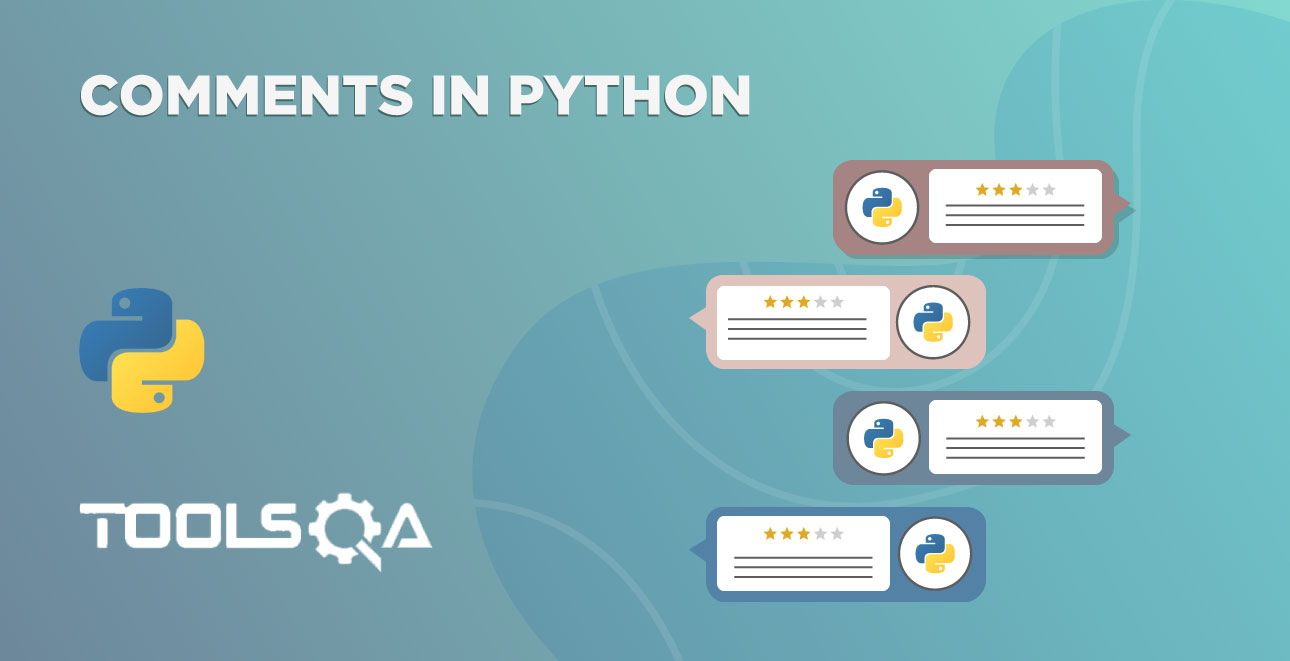We have seen the assignment operator (=), and how we can use it to assign data to variables. Additionally, we have also seen the usage of arithmetic operations. Subsequently, in this tutorial, we will learn a quicker way to do these operations.  We called these shortcut operators. We will discuss in detail:-

• What are Shortcut Operators?

## What are Shortcut Operators?

Let's take an example of variable assignment and increment

count = 1;

count = count + 1;

If we look at this operation, we have got the count variable on both sides of the expression. In addition to that, it is a time-consuming and less efficient way of writing code. Most programming languages have a more efficient way to write this. In the same vein, Python also follows the same.

With Python's shortcut operators, we can write the same expression as

count += 1;

Additionally, we can use shortcut operators on any of the arithmetic binary operators.

The general rule is - If ? is an operator then our long-form will be

variable = variable ? expression

Subsequently, we can write the above expression in shorthand notation as follows.

variable ?= expression

We can place any arithmetic operator in place ?. Let's see some examples in Python IDE.

For example, see the regular operation using the +.

``````# normal operation
count = 0
count = count + 1
print(count)
``````In the statement count = count + 1, we have the same variable on the left the right sides of the operation. So, we can use shortcut variations of the addition operation. Let's see the same example with the shortcut addition operator.

``````# shortcut operators
count = 0
count += 1
print(count)
``````
• The variable count has a value 0 in the first line.
• In the second line, we have added 1 to the count.

What's the output? You can say it as 1. And it's perfect.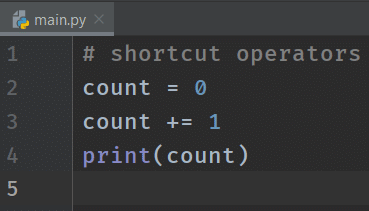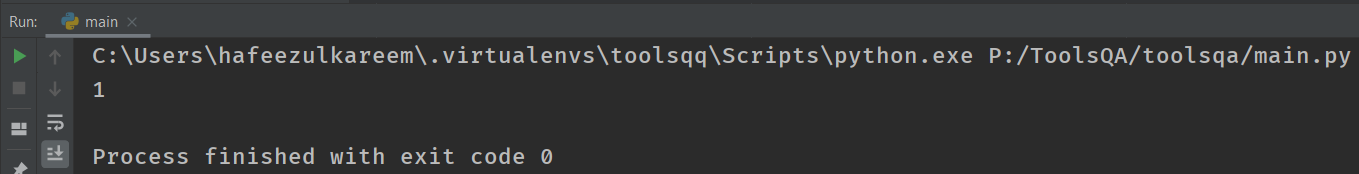If you see the output of the above program and the previous one, both are the same. There is no difference. And this is how we reduce the length of the expressions using shortcut operators.

Similarly, see the following example with the integer division.

``````# shortcut operators
toolsqa = 100
toolsqa //= 2
print(toolsqa)
``````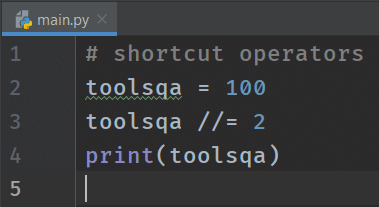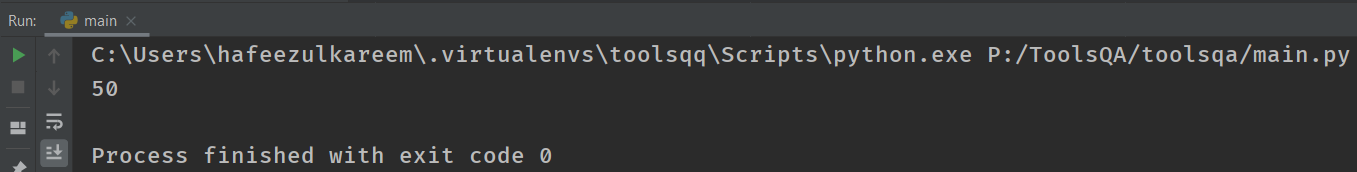We can use all the arithmetic operators as shortcut operators. Here are some more examples

We can represent a = a3 as a*=3*

We can represent b = b/4 as b/=4

## Key Takeaways

• Firstly, we can use the shortcut operator of the assignment if we have the same operand on both sides of the operation.
• Moreover, using shortcut operators reduce redundancy.
• We have all shortcut variations for binary arithmetic operators.
• *Additionally, c = c * 5 can be written as c = 5 in shortcut operation.
• Also, rev = rev + num % 10 =can be written as rev += num % 10 in shortcut operation.

## Shortcut Operators - Practice Yourself

We got a fair idea of shortcut operations; let's practice some code. Remember, practice will make you perfect, so don't skip this section.

#Problem 1

``````val = 0
val *= 10
val += 34 % 10
print(val)
``````

#Problem 2

``````# Try to the write the program by following the below steps
# 1. Initialize the variable "num" with 2 digits number
# 2. Assign the above "num" to a new variable called "original"
# 3. Assign 0 to a variable "reverse"
# 4. Update the value of "reverse" with "reverse * 10"
# 5. Add the result of "num % 10" to the "reverse"
# 6. Update the value of "num" with "num // 10"
# 7. Print "reverse" and "num"
# Note: Use shorthand notation wherever possible
``````

#Problem 3

``````welcome_message = "Hello, "
name = "ToolsQA"
# write the shorthand notation for the following expression
welcome_message = welcome_message + name
print(welcome_message)
``````

#Problem 4

``````num = 10
# write the shorthand notation for the following expression
num = num * (10 // 3)
print(num)
``````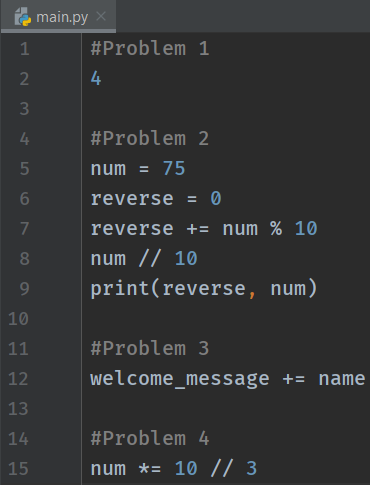## What Next?

To conclude, make sure you have practiced and understood the expressions that are present at the end of the tutorial. Moreover, we will use the shortcut operators frequently in coding. Understand them before going to the next tutorial.

You would have seen the first line of code snaps in each tutorial. Additionally, it starts with a particular character hash (#). What's that, and why do we use it? Let's find the answers in the next tutorial.

Happy Coding :)